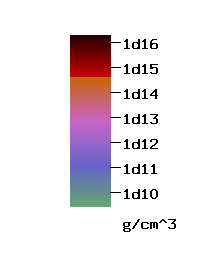X-Z Density Contour in Stellar Collapse after nuclear density is reached

• Mass = 2.47 solar mass (against Max. of spherical star = 1.60)
• Initial Equatorial Radius = 2680 km; Rigid rotation at t=0, T/W=0.0089, q=1.04
• Subnuclear density : Γ=1.3 , Above nuclear density : Γ=2.5; P = Polytropic + Thermal part